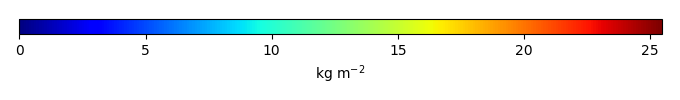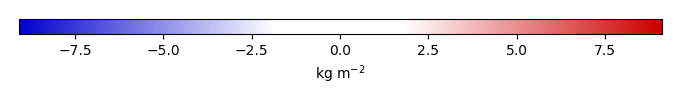# Mean State

Period Mean (original grids) [Pg]
Model Period Mean (intersection) [Pg]
Model Period Mean (complement) [Pg]
Benchmark Period Mean (intersection) [Pg]
Benchmark Period Mean (complement) [Pg]
Bias [kg m-2]
Bias Score 
Spatial Distribution Score 
Overall Score 
Benchmark [-] 32.8
CLM5PHSOFF [-] 47.2 46.4 0.899 32.8 0.0526 3.25 0.604 0.799 0.701
CLM5PHSON [-] 38.5 38.7 0.241 32.8 0.0526 1.73 0.717 0.926 0.822
Period Mean (original grids) [Pg]
Model Period Mean (intersection) [Pg]
Model Period Mean (complement) [Pg]
Benchmark Period Mean (intersection) [Pg]
Benchmark Period Mean (complement) [Pg]
Bias [kg m-2]
Bias Score 
Spatial Distribution Score 
Overall Score 
Benchmark [-] 8.35
CLM5PHSOFF [-] 2.30 1.83 0.177 8.34 0.0114 -1.14 0.433 0.538 0.485
CLM5PHSON [-] 3.24 2.69 0.201 8.34 0.0114 -0.977 0.477 0.622 0.550
Period Mean (original grids) [Pg]
Model Period Mean (intersection) [Pg]
Model Period Mean (complement) [Pg]
Benchmark Period Mean (intersection) [Pg]
Benchmark Period Mean (complement) [Pg]
Bias [kg m-2]
Bias Score 
Spatial Distribution Score 
Overall Score 
Benchmark [-] 10.6
CLM5PHSOFF [-] 10.4 8.62 1.31 10.6 0.0246 -0.228 0.414 0.496 0.455
CLM5PHSON [-] 12.3 11.7 0.363 10.6 0.0246 0.299 0.500 0.561 0.530
Period Mean (original grids) [Pg]
Model Period Mean (intersection) [Pg]
Model Period Mean (complement) [Pg]
Benchmark Period Mean (intersection) [Pg]
Benchmark Period Mean (complement) [Pg]
Bias [kg m-2]
Bias Score 
Spatial Distribution Score 
Overall Score 
Benchmark [-] 4.69
CLM5PHSOFF [-] 3.50 3.38 0.140 4.67 0.0241 -0.393 0.547 0.657 0.602
CLM5PHSON [-] 4.17 4.12 0.0636 4.67 0.0241 -0.0436 0.581 0.798 0.689
Period Mean (original grids) [Pg]
Model Period Mean (intersection) [Pg]
Model Period Mean (complement) [Pg]
Benchmark Period Mean (intersection) [Pg]
Benchmark Period Mean (complement) [Pg]
Bias [kg m-2]
Bias Score 
Spatial Distribution Score 
Overall Score 
Benchmark [-] 27.8
CLM5PHSOFF [-] 78.2 68.2 7.85 26.8 0.921 11.6 0.461 0.886 0.674
CLM5PHSON [-] 39.9 38.2 1.51 26.8 0.921 7.87 0.607 0.919 0.763
Period Mean (original grids) [Pg]
Model Period Mean (intersection) [Pg]
Model Period Mean (complement) [Pg]
Benchmark Period Mean (intersection) [Pg]
Benchmark Period Mean (complement) [Pg]
Bias [kg m-2]
Bias Score 
Spatial Distribution Score 
Overall Score 
Benchmark [-] 43.5
CLM5PHSOFF [-] 32.7 31.7 0.504 43.5 0.0288 -1.30 0.450 0.606 0.528
CLM5PHSON [-] 52.5 51.4 0.326 43.5 0.0288 1.03 0.493 0.626 0.560
Period Mean (original grids) [Pg]
Model Period Mean (intersection) [Pg]
Model Period Mean (complement) [Pg]
Benchmark Period Mean (intersection) [Pg]
Benchmark Period Mean (complement) [Pg]
Bias [kg m-2]
Bias Score 
Spatial Distribution Score 
Overall Score 
Benchmark [-] 32.0
CLM5PHSOFF [-] 27.8 25.6 2.06 31.9 0.0804 -0.408 0.571 0.852 0.712
CLM5PHSON [-] 31.6 31.8 0.632 31.9 0.0804 0.262 0.606 0.861 0.734
Period Mean (original grids) [Pg]
Model Period Mean (intersection) [Pg]
Model Period Mean (complement) [Pg]
Benchmark Period Mean (intersection) [Pg]
Benchmark Period Mean (complement) [Pg]
Bias [kg m-2]
Bias Score 
Spatial Distribution Score 
Overall Score 
Benchmark [-] 21.1
CLM5PHSOFF [-] 32.8 28.1 2.82 20.9 0.146 1.00 0.524 0.714 0.619
CLM5PHSON [-] 24.8 23.3 0.610 20.9 0.146 1.28 0.600 0.820 0.710
Period Mean (original grids) [Pg]
Model Period Mean (intersection) [Pg]
Model Period Mean (complement) [Pg]
Benchmark Period Mean (intersection) [Pg]
Benchmark Period Mean (complement) [Pg]
Bias [kg m-2]
Bias Score 
Spatial Distribution Score 
Overall Score 
Benchmark [-] 3.81
CLM5PHSOFF [-] 5.61 5.80 0.158 3.81 0.000319 0.574 0.531 0.780 0.655
CLM5PHSON [-] 6.88 7.17 0.0941 3.81 0.000319 1.03 0.512 0.754 0.633
Period Mean (original grids) [Pg]
Model Period Mean (intersection) [Pg]
Model Period Mean (complement) [Pg]
Benchmark Period Mean (intersection) [Pg]
Benchmark Period Mean (complement) [Pg]
Bias [kg m-2]
Bias Score 
Spatial Distribution Score 
Overall Score 
Benchmark [-] 9.16
CLM5PHSOFF [-] 18.3 14.3 3.29 8.87 0.289 0.648 0.585 0.781 0.683
CLM5PHSON [-] 11.0 10.0 0.829 8.87 0.289 0.760 0.605 0.812 0.708
Period Mean (original grids) [Pg]
Model Period Mean (intersection) [Pg]
Model Period Mean (complement) [Pg]
Benchmark Period Mean (intersection) [Pg]
Benchmark Period Mean (complement) [Pg]
Bias [kg m-2]
Bias Score 
Spatial Distribution Score 
Overall Score 
Benchmark [-] 12.0
CLM5PHSOFF [-] 21.3 18.6 2.16 12.0 0.0529 1.14 0.418 0.353 0.386
CLM5PHSON [-] 18.2 17.4 0.537 12.0 0.0529 1.77 0.466 0.349 0.407
Period Mean (original grids) [Pg]
Model Period Mean (intersection) [Pg]
Model Period Mean (complement) [Pg]
Benchmark Period Mean (intersection) [Pg]
Benchmark Period Mean (complement) [Pg]
Bias [kg m-2]
Bias Score 
Spatial Distribution Score 
Overall Score 
Benchmark [-] 368.
CLM5PHSOFF [-] 543. 500. 43.1 363. 4.64 1.09 0.501 0.698 0.599
CLM5PHSON [-] 487. 477. 9.77 363. 4.64 1.46 0.573 0.784 0.679
Period Mean (original grids) [Pg]
Model Period Mean (intersection) [Pg]
Model Period Mean (complement) [Pg]
Benchmark Period Mean (intersection) [Pg]
Benchmark Period Mean (complement) [Pg]
Bias [kg m-2]
Bias Score 
Spatial Distribution Score 
Overall Score 
Benchmark [-] 1.24
CLM5PHSOFF [-] 0.978 0.599 0.280 1.23 0.00783 -0.137 0.417 0.661 0.539
CLM5PHSON [-] 1.55 1.22 0.341 1.23 0.00783 0.0659 0.507 0.738 0.623
Period Mean (original grids) [Pg]
Model Period Mean (intersection) [Pg]
Model Period Mean (complement) [Pg]
Benchmark Period Mean (intersection) [Pg]
Benchmark Period Mean (complement) [Pg]
Bias [kg m-2]
Bias Score 
Spatial Distribution Score 
Overall Score 
Benchmark [-] 5.62
CLM5PHSOFF [-] 11.6 10.9 0.500 5.59 0.0265 0.893 0.448 0.476 0.462
CLM5PHSON [-] 14.5 14.1 0.228 5.59 0.0265 1.60 0.345 0.405 0.375
Period Mean (original grids) [Pg]
Model Period Mean (intersection) [Pg]
Model Period Mean (complement) [Pg]
Benchmark Period Mean (intersection) [Pg]
Benchmark Period Mean (complement) [Pg]
Bias [kg m-2]
Bias Score 
Spatial Distribution Score 
Overall Score 
Benchmark [-] 8.21
CLM5PHSOFF [-] 16.3 13.8 1.71 8.09 0.122 1.71 0.464 0.481 0.473
CLM5PHSON [-] 10.2 9.46 0.474 8.09 0.122 1.74 0.539 0.599 0.569
Period Mean (original grids) [Pg]
Model Period Mean (intersection) [Pg]
Model Period Mean (complement) [Pg]
Benchmark Period Mean (intersection) [Pg]
Benchmark Period Mean (complement) [Pg]
Bias [kg m-2]
Bias Score 
Spatial Distribution Score 
Overall Score 
Benchmark [-] 14.8
CLM5PHSOFF [-] 7.11 5.93 0.824 14.8 0.0147 -2.14 0.495 0.767 0.631
CLM5PHSON [-] 12.0 11.7 0.182 14.8 0.0147 -0.425 0.652 0.870 0.761
Period Mean (original grids) [Pg]
Model Period Mean (intersection) [Pg]
Model Period Mean (complement) [Pg]
Benchmark Period Mean (intersection) [Pg]
Benchmark Period Mean (complement) [Pg]
Bias [kg m-2]
Bias Score 
Spatial Distribution Score 
Overall Score 
Benchmark [-] 20.1
CLM5PHSOFF [-] 32.6 31.8 0.793 19.8 0.219 2.14 0.447 0.651 0.549
CLM5PHSON [-] 43.6 43.6 0.247 19.8 0.219 4.91 0.403 0.721 0.562
Period Mean (original grids) [Pg]
Model Period Mean (intersection) [Pg]
Model Period Mean (complement) [Pg]
Benchmark Period Mean (intersection) [Pg]
Benchmark Period Mean (complement) [Pg]
Bias [kg m-2]
Bias Score 
Spatial Distribution Score 
Overall Score 
Benchmark [-] 8.04
CLM5PHSOFF [-] 1.45 1.37 0.182 7.99 0.0515 -1.66 0.414 0.254 0.334
CLM5PHSON [-] 3.04 3.04 0.0352 7.99 0.0515 -1.10 0.511 0.490 0.500
Period Mean (original grids) [Pg]
Model Period Mean (intersection) [Pg]
Model Period Mean (complement) [Pg]
Benchmark Period Mean (intersection) [Pg]
Benchmark Period Mean (complement) [Pg]
Bias [kg m-2]
Bias Score 
Spatial Distribution Score 
Overall Score 
Benchmark [-] 88.9
CLM5PHSOFF [-] 150. 147. 1.87 88.8 0.0655 6.82 0.534 0.778 0.656
CLM5PHSON [-] 129. 128. 0.373 88.8 0.0655 5.00 0.646 0.945 0.795
Period Mean (original grids) [Pg]
Model Period Mean (intersection) [Pg]
Model Period Mean (complement) [Pg]
Benchmark Period Mean (intersection) [Pg]
Benchmark Period Mean (complement) [Pg]
Bias [kg m-2]
Bias Score 
Spatial Distribution Score 
Overall Score 
Benchmark [-] 9.09
CLM5PHSOFF [-] 20.5 19.6 1.02 9.08 0.0128 1.50 0.352 0.257 0.305
CLM5PHSON [-] 21.8 21.8 0.283 9.08 0.0128 2.13 0.323 0.298 0.311
Period Mean (original grids) [Pg]
Model Period Mean (intersection) [Pg]
Model Period Mean (complement) [Pg]
Benchmark Period Mean (intersection) [Pg]
Benchmark Period Mean (complement) [Pg]
Bias [kg m-2]
Bias Score 
Spatial Distribution Score 
Overall Score 
Benchmark [-] 2.45
CLM5PHSOFF [-] 8.11 7.87 0.449 2.40 0.0496 1.82 0.372 0.271 0.321
CLM5PHSON [-] 6.26 6.15 0.140 2.40 0.0496 1.92 0.352 0.318 0.335

# Temporally integrated period mean

BENCHMARK MEANMODEL MEANBIASBIAS SCORESPATIAL TAYLOR DIAGRAMMODEL COLORS# Spatially integrated regional mean

MODEL COLORS# All Models

BenchmarkCLM5PHSOFFCLM5PHSON